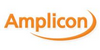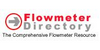### OctalThe octal numeral system, or oct for short, is the base-8 number system, and uses the digits 0 to 7. Octal numerals can be made from binary numerals by grouping consecutive binary digits into groups of three (starting from the right). For example, the binary representation for decimal 74 is 1001010, which can be grouped into (00)1 001 010 – so t...
Found on http://en.wikipedia.org/wiki/Octal

### octaladjective of or pertaining to a number system having 8 as its base; `an octal digit`
Found on https://www.encyclo.co.uk/local/20974

### OctalA number system using the base-8, i.e., each digit can be any of 8 values, represented by the digits 0-7. Each octal digit can also be represented by a three-bit binary number. See also: Binary Notation.
Found on http://www.diracdelta.co.uk/science/source/o/c/octal/source.html

### OctalA numbering system which uses a base of 8. The symbols used to represent a numerical value are 0, 1, 2, 3, 4, 5, 6 and 7. For example the value 23 decimal is 27 octal
Found on http://www.amplicon.co.uk/info/glossary.cfm

### OctalPertaining to a base 8 number system.
Found on http://www.flowmeterdirectory.com/flowmeter_technical_glossary/flowmeter_te

### octal[adj] - of or pertaining to a number system having 8 as its base
Found on http://www.webdictionary.co.uk/definition.php?query=octal
No exact match found